WAVE COORDINATES

ATOMIC  MOLECULAR  MAGNETIC  GRAVITATIONAL

CHAPTER 55INDEX TO OTHER PAGES

1. In order to enhance the, "how to", in gravitational descend from the previous page, we need to understand a bit more on the coordinates of magnetic lines and wave formations.

2. One of the first things we should mention is, how enormously perfect, and for us no doubt complex, the atom, and molecules as well are.  Their complexity in - what is best understood by "angular coordinates", is beyond our understanding.

3. It may appear simple to the many of us whose knowledge of fundamentals is rather elementary, but if it is not absolutely grandiosely marvelous you have a long ways to go in comprehending nature.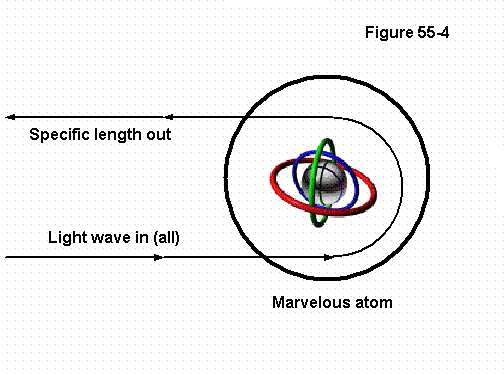Figure 55-41. Just consider how sunlight enters upon the green leaf of a tree in all lengths thereof, and coming upon the atoms and molecules of their compound form, all but the green wavelengths are as we may say "arrested", the green lengths alone leave it to come into our eyesight.  (Figure 55-4 serves to project some illustration)

2. What therefore is in these atoms or molecules to perfect that most marvelous feat?  It certainly is not a die, but rather each single atom for its product is movement, angular movement, which it shares with other atoms to the tune of molecules in the form of coordinates, their angular movements coordinating with each other.

3. And in so doing each different element has its own signature, which is in coordinates by what comes to be known as wavelengths.  How thus by all these many parts in their movements, do these coordinates operate so perfectly, to be so unique in so many untold ways?

4. We can alter the wavelength of Sodium and sending it back through, it recognizes its signature immediately.  If we send sunlight through glass or water all of it will pass on through, but upon our skin, it comes to a halt, and while doing so it warms us by what I came to call RAM (other subject).

5. The air for example mostly nitrogen gas, appears transparent, but that is only so because the light finds no connection in its molecules, while the water-vapor in the clouds do and reroutes all such wavelengths as it "recreates" down to our eyes. For it does not pass the originals from the sun to us lest we would see the sun in the clouds, just as you would when sunlight is reflected by a mirror.

6. When you see a bird in the sky it is because the particular wavelengths recreated in all the colors of its body are send out to all directions.  Wherefore it matters not where you stand the bird is visible to all, but the same entails that these molecules in their atomic coordinates are radiating these wavelets to all directions from a single incoming direction.

7. Take water for example, the element H2O, a mere three atoms that are well bound to each other, but associate loosely with all others of its kind to be a liquid. But with a little RAM, that is to say - heat into it, these begin to demand a lot more room becoming something between gas and liquid.

8. And they are very determined in their greater spacing as we know from the pressure of steam. And how does this all work?  They do not stick together with glue, nor are there arms and legs, nor intermediate matter, nor poles or strings, since their product as well as their existence is movement in coordinate formations.

9. In some fashion I know their principles in operation, but I cannot begin to lay out or define the real how so in these marvelous things. That which I therefore can relate to you are the versions in principle, which all in itself is already marvelous if only I could find the words to properly express my thoughts.

1. We wanted to know more about this gravitational something that is more than just a single atom or gyro by which things are brought down to earth. While therefore we all know how and why a gyro acts as it does, just as we think to know what an atom is and how it works, when we dig deeper it becomes far more complicated.

2. And like molecules in compound form show to be integrate elements or components of nature, so the fundamental components of magnetic and of gravitational descend become far too intricate for me to lay out in full detail.

3. By figure 55-1, I drew what in my mind is the most realistic schematic of the principle by which these operate. I discussed the magnetic component in a previous page which as illustrated shows the means by which linear magnetic lines lay hold of the media in its heavier metallic form.

4. It is to show that the so called lines of power that draws things are not single, nor pass merely by single atoms, but rather that the "mean" direction of movement in the way of power is by the whole in one direction.

5. Then coming to the gravitational component (to call it that) these can however be in the form of fewer atoms in lighter media, as well as by greater number in that media which is the heavier mass.  This version too in design acts similar that figure of eight that is so fundamental of that grandiose force called magnetic.

6. In the drawing above I drew it in the state of normal, the imaginary axis being at full right angle to the 3M, or magnetic lines.  Here too - while these atoms and/or molecules work in unison with one another -- the pattern in their coordinate is very much like we observe on a single gyro.

7. From which also the "mean" direction is not after the basic 3M, but into their specific direction in their own coordinate, which of-course is again born forth and en-powered by the main 3M.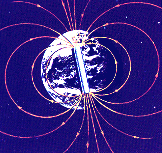1. For here again in looking at the illustration of the earth with its magnetic lines, and we ascribe the outgoing movement to the North with the ingoing to the South,  "that" movement in the direction thereof is the "mean" direction of the force.

2. The 3M in themselves are into both directions in and out at both poles, only they can not register themselves as such by the coordinate on which they become of power.

3. Just as it is with atoms that by themselves are mere elements not in compound form, so it is with fundamental movements. And if a single person cannot transport a log, a dozen men can, and better yet when coordinated means are employed.

4. Our scientists are good at making models and illustrations of compound structures, and how these by variations can be made more rigid or pliable, or to serve in different ways under different circumstances, but to explain how these many parts fundamentally interact with each other to do so, that is a very tall order.  All this because there is no glue nor rubber bands, but simply movement in coordinates.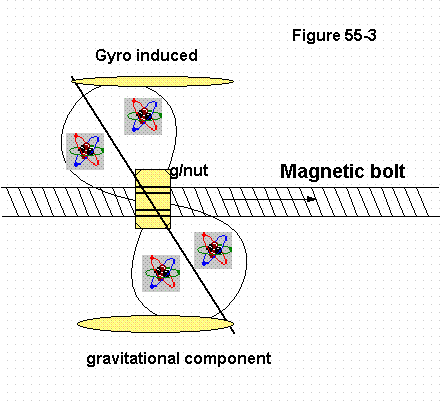Figure 55-3 click to enlarge

1. Figure 55-3 then is to illustrate the gravitational component when it is "out of the normal", so known when there is a torque upon its angular inertia.  And as mentioned before this may very well be likened to a nut in precessional rotation bearing down upon the magnetic lines of movement.

2. The velocity at which this process enhances itself has come to be known as the "acceleration of gravity in the value of 32.14 ft/sec/sec. (sea level)

3. The nut in fact gains speed in the linear as it increases in rpm.  If we take the linear value of the velocity of the lines (300.000 km/sec)  and divide it by the 32.14/ft, the nut gains speed each second of time.  We might thus conclude that it could come to a downward velocity of 9334 km/sec or by the square to 290 km/sec.

4. But if this is correct, or anywhere near so,  I do not know.  We do know however that the downward rotation is not in volume as mass or weight, where the heavier object would fall faster.  This then should be logical since it is procured by a precessional torque.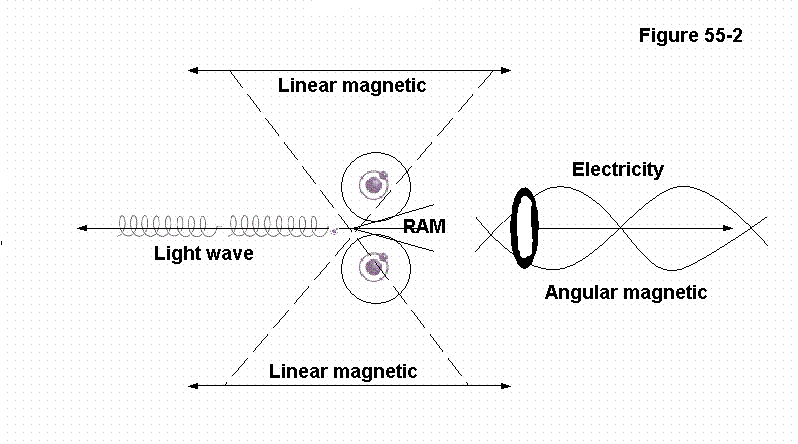Figure 55-2 click to enlarge

1. Then let us proceed to some derivatives of the magnetic spectrum that our men of the past came to call, "the electro-magnetic spectrum".  (Figure 55-2).  That spectrum then is not of electric seeing how electric is but an derivative, or cousin of magnetic, just as radio waves and all other waves are.

2. Electricity is of course not a wave, but the closest cousin to the main force,  the main being termed linear, with electricity the angular magnetic cousin.

3. Then in coming to light in its wavelengths, where I formerly said, all we have to do is to snip one of these magnetic lines to bear it forth, the reality is a bit different.

4. Light-waves now also are not just wavy lines, but circular formations that pass around the perimeters of atoms.  Each turn in their formation is their wavelength, the more that (so called) spring is expanded the longer the wavelength, and shorter when more compressed.

5. How therefore do we generate these wavelengths of light?  In all reality it is not molecular (like radio waves etc or except perhaps very dim,) but rather on the atomic order.

6. For this we need RAM, which is spoken of on page 21 of this website. RAM stands for "relative angular movement" which in itself is "not" a movement of angular, but the relation between two or more angular movements.

7. We can also look at it as if beating on, or shaking an element such as Tungsten to raise the relative angular moment of its components in unison to a state that they seem to be vibrating or at war with one another, and turning red hot or even white hot.

8. This is something we do every day by driving an angular magnetic force (electricity) into the tungsten, our common household light-bulb.

9. RAM then to reiterate it a bit more is like - "a change of". Consider the atoms and molecules of Tungsten to be at room temperature with all its internal angular moments and movements happily balanced (so said). Then by sending an electrical current through it, we are so to say driving them crazy, upsetting them in their happily balanced state.

10. This however is not in the way of causing them to vibrate at the core since that is the essence of sound, in which case we should hear a lot of noise when turning on a light.

11. To find the words for it, look at the illustration where I wrote RAM how I placed it between the two angled lines, one of each atom, the lines convey the angular movement of each, and the RAM is the "relation" between them, as in "that state of being".

12. The angular movement of them being at a difference with one another, also making for "changes" which if not protected would rip them apart.  This is "heat", or the essence of it, and the term oscillation can also be used provided we apply it to angular movement rather than material components.

13. The oscillations then is what provides the light. And to provide us with some idea how this proceeds, consider a lathe in which there is a round block of aluminum turning a high rpm. Then consider the cutting tool as it scrapes off strings of material which fan out in a circular fashion.

14. We might say that generating light is like chapping on a wheel. The specific movement of the compound (Tungsten in this case) is angular, with the RAM; not in itself being, but providing the cutting tool.  The resultant then is again an angular driven away into the linear, hence our wavelength of light.

15. Radio waves and most other waves are generated in much the same fashion utilizing oscillators.  And even when we generate electricity by the rotation of the armature we are twisting and turning the magnetic lines of force so as to preserve them upon a guide, a so called wave guide that is nothing more than a metal wire.

16.  Here comes a question often on the minds of man, a question to which there is an answer, but not such as may be given, it being "What is magnetism?"

17. And now I have this problem that while I am defining fundamentals speaking of these things how in my judgment I am essentially using, and yes how shall I put this, to say "much too elementary terms", almost like utilizing the wrong terms, since I must stay within boundaries.

18. Wherefore again to the quest as to what magnetism is, or the fundamental scope of the atom, accept it as movement, and leave it at that since again in all essence I must refer you to "Spectacular," and what I pronounced there,

GRAVITATIONAL  INERTIA

1. Thus far I have written seven pages dealing with gravity and the cause thereto, and in the eight page I came to simplify  the relation of force to media.  Then I questioned myself why with all this definition may it still be so hard to comprehend?

2. And so I am asking my reader: by these sentences here below - do you indeed understand what is meant thereby, and the process in how and why these become valid?

3. "One cannot be weightless lest all of his weight is exceeded into the (his) inertia of velocity."

4. "Gravity will draw upon all mass according to its first inertia by a factor limited to +/-32 lb." (sea level)

5. "Gravitational force being a product of movement by movement into movement."

6. I have seen how deeply man is in ignorance towards the cause and nature of this force, and I realize it was not for him to discover it, but some of the fabrication thereto is astounding, pitiful indeed.

7. And I sigh for man, not so much for him not knowing - but his resentment against anything new in that in which he cannot, or refuses to lay a hold upon.

8. So I reasoned, new wine is for new wine-skins, the old will not accept it for they are contend with their wine that is no wine. Whom therefore will I be speaking to that will hear, and have I presented it sufficiently clear for these new and younger ones to grasp?

9. And so I thought to do this once more this time placing the emphasis upon the mathematics in the inertia to do justice to the cause.

10. Graphics are always helpful, so let us take this slowly one step at the time. To the left of the illustration, at the equator, we have a weight of 165 lb. This is an object of mass, of material that on the scale shows 165 lb, and we have universally accepted that - weight in lb (or kg, etc) is the amount of g/force. This weight then at earth's equator is some 4000 miles from its center wherefore there is a centrifugal action upon it as well.

11. The amount of that centrifugal by still another universal law that we have accepted comes to 18.24 lb.  (see example 1).  Then again by the universally accepted law of equal action to reaction, the factual total of g/force on the object of spring (scale) weight 165 lb, is the sum of them to 183.24 lb.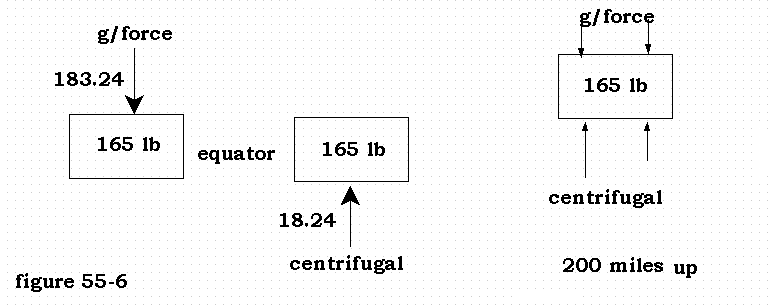Figure 55-6

Example # 1.  165-lb x 1521-ft/sec2 : 20,929,920-ft/r = 18.24-lb

1. Next, we want that weight to be free floating from the surface of the earth, we wish to defy or overcome that g/force. With a toy gyro this is simply by spinning it, but that being of the angular movement it will defy the g/force only in the horizontal, not in the vertical as we wish with our weight so illustrated.

2. In order therefore to fulfill our wish what we need to do is to "add" "centrifugal" impact to it, since by law any g/force may be offset by an equal amount of centrifugal to be free floating, or weightless as it may be said.

3. For our demonstration then we will keep adding centrifugal to our object until it floats free which will be at 200 miles above the earth's surface, (we do not want to cause any accidents with our high speed so close to the surface).

4. Our object now already has a centrifugal upon it to the tune of 18.24 lb, and what we need is a total of  183.24 lb, (the centrifugal to equal the g/force). All we need is 165 lb of centrifugal to add.  And the way to do it as we know is by adding more velocity to the object.

5. How simple therefore this will be, wherefore let us bring the object from its current 1000 mph to the speed of 6908 mph that is 4710 ft/sec and should come to 165 lb. of centrifugal.

6. Accordingly; 165 lb x 4710 ft/sec/sq : 22,176,000 ft/r = 165 lb

7. The radius given is of course the 4200 miles from earth's center. But will our object stay up there at a speed of no more than 6908 mph?  Our quest is, now that we found that 165 lb of centrifugal to counteract that g/force as we surmised -- are the two forces equal so it may float free for us?  The answer is; "No, it will not.

8. But why not? For then we can reason, well we gave it the centrifugal equal to the g, and that linear velocity we placed upon it, if anything - the two should be equal.

9. And yes it would be so except for one snag, namely; that velocity does not double but squares the force.  And that "Gravity is implemented by movement, and "centrifugal augments gravitational". Which in essence is as inertia producing gravity.

10. A mouth full, is it not? And to fully grasp this we must think clearly and consider the following:  The weight in its g/force is a great number of atoms producing an angular momentum, and this momentum has the ideal of attempting to stay put, or be weight for us, to resist us, or resist movement.

11. When therefore we insist upon it to move by a linear path, to add velocity, it becomes even more fixed in place, presenting an even greater degree of resistance.

12. For here we have two "inertia's" the angular of the mass, and the linear we placed upon it.  But is the linear really an inertia in itself?  It is speed yes, but speed of what, or upon what?

13. It is the speed upon the existing angular, and as such compounds that angular.  Noting again therefore that the linear velocity even though we call it an inertia, is not in itself so - but its factor upon the angular inertia.

14. Consequently, in adding that velocity to come to the extra 165 lb in centrifugal, that very same velocity began to compound our 165 lb of inertia to a greater and greater sum, and we had to keep on going in our velocity to accelerate it more and more to somehow arrive at a point where the one would leave off for the other to come to an equal proportion.

15. Up to now we do not have a law of gravitational force, the one so called by it - here below - is factually of "Centrifugal" since it computes centrifugal.  This is:   "The gravitational acceleration of a mass in  uniform circular motion is proportional to the square of its speed and inversely to the radius of its path".

16. In proper wording it should read: "The centrifugal resistance of a weight in  uniform circular motion is proportional to the square of its speed and inversely to the radius of its path".

17. If then it seems that I am splitting hairs, I beg to differ, since yes the figure of gravitational would be the same for centrifugal since ""conveniently"" the two always equal. At least so it is said, but that also is not true in itself.

18. And yes the one must equal the other for our object to be free floating, or as it may be said;  "be fixed in orbit",  but when not fixed in orbit the two are never equal, or how else would the object go up, or the astronauts come back to earth?

19. What there is left for us to do is: - to write a law of gravity, and not just a law, but the correct law of gravity.  We now know that Newton's law of gravity was in error, and why then, by the way, are we still writing gravitational in his law of centrifugal?

20. The correct law of gravitational force then is: "The gravitational force upon a mass in uniform circular motion is; its (earth) weight times the value of g at the radius thereof."

21. For here if we first calculate the centrifugal by Newton's law (example 2)  it comes to more than 5000 lb instead of the 165 lb we came to add first.  That mind you is a lot of force over and beyond where we started at 165 lb.

22. The reason for this as we should surmise, lies in the "square", how we have to square the force in velocity of the linear component, since it is in that degree that the linear compounds or augments the angular.

23. Example # 2.  165-lb x 26400-ft/sec2 : 22,176,000-ft/r = 5,186-lb/ : 22,176,000-ft/r = 5,186-lb/c (centrifugal)

24. Now let us -- by our new law -- calculate the force of gravity on an object of 165 lb that we placed 4200 miles from earth's center.  While g is around 32.14 at base or sea level, it is some  31.43 lb at the new radius, which is about plus one percent decrease, thus:  165 lb x 31.43 = 5186 lb/g  (gravity - force of)

25. This law is truly a law of gravity since we are computing g/force by g/force rather than centrifugal to conveniently apply it to gravity as well.  (Or, inertia by inertia) And better said; We are computing g/force by the 'factor' of g/force.

26. Then of course we can take the same convenience in our new law to centrifugal.  But whenever the figures do not jibe one force always exceeds the other.

27. But let us have another good look at our law of gravity; how its weight that in itself is g/force comes to multiply by g/force in the factor thereof.  The secret is in this that "inertia is multiplied by inertia" (the angular by the linear -- the object of mass in linear or uniform circular motion.)  {Or: Gravity by the factor thereof.}

28. Then there is another thing we must get away from to thrash it altogether, namely; how uniform circular movement is a movement of constant, and not falling into acceleration.  To insist upon it certainly is not wisdom nor knowledge, but foolishness.

29. The acceleration of gravity is said to be 32 ft/per second per second.  But that is only correct near the surface of the earth where the factor of gravity is 32. (rounded off) Two hundred miles up where the factor of gravity is 31.43, the acceleration of gravity is 31.43 and not the 32 near the earth's surface.

30. And if one recedes far enough into space where the factor of gravity becomes 28, the speed at which an object will fall from that height is 28 ft per second per second. But as it comes closer and closer to the earth the speed increases to remain in tune with whatever the factor of gravity is at the various altitudes, increasing to 32 when at sea level.

31. Then if one can answer me how and why at greater distances from the earth the objects accelerate slower, and your answer is fundamentally correct, I am pleased in that you are indeed learning from what I have placed before you.

32. The downward movement of all substance under the influence of gravity does not travel according to its weight, nor according to mass, but according to the factor imposed upon its inertia, the factor that bears upon the precessional inclination thereof.

33. That factor then is expressed numerically found by the acceleration thereof, since clearly it is the spiraling movement of all inertia drawn by the magnetic lines of force of any sun or planet.  (not fair is it, to give you a hint?)

THE FACTOR OF INERTIA

1. Let us enhance this factor of inertia, or of gravity as it may be called while utilizing a graphic image.  Generally man looks upon the figure of 32.174 in the manner of feet per second, per second, in the velocity by which things accelerate downwards by the force they call gravity.

2. The Almighty Lord and Creator did not however furnish man with any insight therein lest he would also come to know the very foundations of the earth, which is but for the Lord Himself to reveal to mankind lest man would die the death of all eternities, and never come to live.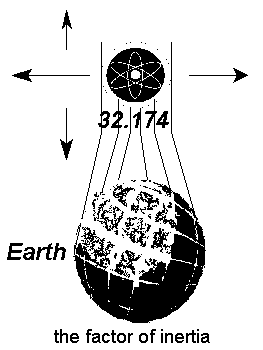1. Allow me then to reiterate that the object above the earth in the illustration, is a mass fully made up of many atoms, that in effect act as gyro's, and as such become an inertial mass. Meaning, it has rigidity, by the mass of movement that constantly returns to where it came from, (turning a circle) and as such affixing itself in space, to become a fixed object inhibiting a resistance towards movement in the linear.

2. (Another example of how going in a circle is not necessarily a change in direction of.)

3. This particular resistance inhibiting the mass may then be given a value of, to for example say, that it has an inertial value of 55 in the way of pounds to force.  But why should it be arbitrarily set at 55, or any other number, when for its measure in a downfall, or gravitational draw per second of time it travels 32.174 ft per/second?

4. For our mathematics is it mandatory that this inertia be given a value of, and what better figure to use than that measure in feet, as also the measure for its resistance in pounds of force.

5. Thus that figure of 32.174 has a twofold use both in ft, as well as in lb of force, and for a final or third use, simply as that figure without the utilization of ft, or lb, to use as a, or the factor in the means of mathematics, the coordinate if you will.  How then is this figure of 32.174 at sea level so correct in every way?

6. And by what, in what way does it come to that?  As the lines in the illustration are to demonstrate, it is the earth by its force, and in its composition, as well as in its movements that place that particular value upon that mass. And I am strictly speaking of mother earth, and no other sphere.

7. If thus we take that mass and let it fall down it effects the factor of 32.174 in an ever increasing velocity.  And when we move it up, it will resist our movement by that same factor when as such we come to refer to it in pounds of force or resistance.

8. Then as we move it sideways to any direction there appears to be something peculiar about it, for while at all times we associated that measure exclusively with the force of gravity, it comes to resist us in exactly the same measure when in a direction of movement that has no bearing whatsoever on gravity.

9. When you take a turn on the road - your inertia in the linear of your speed coupled with the angular inertia of the mass at its factor of 32.174 tends to keep you fixed in a straight line, and you must endure its resistance to change in what is called the centrifugal impact upon you.

10. So thus how and why is that factor the same for any turn in the horizontal as it is for gravity in the up or down? Does not the suggestion point us to it being a factor of "inertia" as well, or rather a factor of inertia more than that of gravity?

11. The answer lies in both, it is a factor of inertia that is born forth by a combination of multiple factors.  The inertial value of the mass as shown in the illustration need not be 32.174, as in fact it rates that particular value only at sea level on mother earth.

12. It may just as well be a value of 6, or of 55, as in fact we saw how 200 miles above the earth its value had decreased to 31.43.  And on the surface of the sun the value is higher than 32.174.

13. It is therefore common sense to realize how that factor of gravity and/or inertia, as we know it here is inbred in the earth. I now did not say - in the forces of the earth, but simply earth, since as I mentioned before, coupled with earth's force - is its composition, as well as all of its movements that in unison procure a specific inertial value upon all the media around it.

14. And as we depart from the body of the earth, that value decreases. When we are on the moon, where the force of gravity is less than what we experience on earth, the inertial factor is likewise less, and the acceleration in a downfall is equally slower.

15. When the earth imposes an inertial value of 32 upon a mass, it will draw upon that mass in a downfall that per feet in seconds of time is equal that particular value of 32 in the torque upon the precessional inclination of the mass, consequently it accelerates by 32 ft p/sec p/sec.

16. When therefore the factor decreases, so does the torque, and consequently the acceleration in feet per second.

17. How therefore with this truth of our Almighty Creator before us, are we to continue in our childish way to say that objects draw to each other according to their mass, and distance between them - seeing how the inertial (or gravitational) value varies from body to body, and from altitude to altitude, and is effective according to the movement, the forces, and the nomenclature of each and every different body?

RETURNING TO OUR SUBJECT

1. This now my dear reader "IS" gravity, the very calculations in themselves prove it.  And they prove something else, namely; how the relevance and or power, increase and/or decrease (of gravity) is by "MOTION" .  Or must I reiterate how inertia, angular, and linear is in fact motion augmenting gravity, since again it certainly is not rice, nor cabbage, nor the wind under it.

2. Motion then is motion the like, for the first 18.24 lb of g/force and centrifugal upon our 165 lb object was by the 1000 mph of mother Earth going for its daily spin, the additional 17000 mph we placed upon it was by our devices.

3. While the value of the fundamental inertia (+-32 lb) towards a downward pull is inbred with the earth in all of its relative movements orbital as well as galactic together with the specific strength of the earth's magnetic entity.  For this reason this particular +-32 lb is for this earth, and not necessarily of any other sphere.

4. If by example linear inertia would merely multiply rather than square itself in force, we would have a short or long way to come even with the force at which gravity will exert itself.

5. For as we have seen we must place enough  inertia by the linear upon the angular speeding towards its orbital path so that it will (as we might say) outruns it inertial value of force.  which by our example was roughly 98 to 99% of what the value of g/force is upon mother Earth.

6. Gravity therefore in the factor thereof is limited, (By example) at 200 miles above the equator it cuts off at 31.34 (in Lb) after which it can no longer retain the object in its greater centrifugal inertia, wherefore also it may depart for greater distances from the earth.

7. And now that we are this far let us once again reiterate the values of g as it applies in different situations.  In finding a force of gravity we do not bother to name nor utilize this sec/by/sec so correct for an object in free fall in a state of acceleration.

8. Nor do we need to add the term pounds etc behind the numerical value, when we compute the various calculations.  Only if we are going by kilograms we of course must use the kilogram value of g as well, or if in pounds as we did here, the numerical would be as here stated.

9. Then again to bring to realization how well gravity will hold onto all substance until it runs out of its own power, consider the great difference in power between the 165 lb all the way to more than 5000 lb.

10. As therefore that angular inertia is so greatly multiplied even so the "torque" upon its axis (to bring it down by magnetic interaction) is multiplied, and yet by its g/force in fundamental scope (the 31.34) which by comparison in pounds seems small, will be overcome.

11. The reality here is that the 31.34 is the "factor" of it, not the actual force or power, or resistance or inertia, however we wish to term it. For is not the 31.34 the factor of each of the 165 individual pounds of the object?  Therefore also when multiplied gravity reveals gravity, or gravity compounds its own inertia.

12.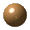Ok now, I think I done my best to make the foundations of the earth appear as simple as possible, and I am going to quit playing with it.  For in all reality we have come to comprehend the very foundations of the earth.

13. Since gravity along with her magnetic husband is, or are as such, the pillars upon which the earth rests.  There are no concrete bases, nor steel girders, but like every other planets so it is held and moved in space.

14. And now that you know - speaking to the whole of the world, to every single person now or to come, do not, and I repeat, "do not" impute this knowledge, that is to say its discovery into me.  You will only make me angry in doing so.

15. It was by God's mercy that now you know, and by His instructions, by my Father I received in order that I may pass the same onto you.

16. And for those who do not believe in my Father, by whose will I came, nor in His Son by whom we have life, you are indeed to be pitied, and most ignorant so I must say - not to see the grandeur of His works in the things that are made.

17. {Weight (standard) as a measure of gravitational force is that which it comes on the scale.

18. Weight in g/force for its full measure on an object resting on Earth is its weight plus the centrifugal impact upon it.}

My toy box, - The foundations of the earth

1. It is a mess the way this so called book I wrote of science and physics is organized, and I wish to tell you how and why it came to that. These pages here were not written at any one time, nor in specific order, but over a period of years, since my age of 24.

2. And when it comes to physics or the sciences I am like a child here a little there a little.  It is not my profession nor my main interest, but rather a sideshow like unto a toy to play with.  And at times many years passed before I wrote again something.

3. When I started however I never thought it would come to the volume that it is now, and while I wrote out so called chapters, I sometimes followed up on it with something on the order of a short article for possible publication.

4. And so in putting it all together it became mixed up with some of it as duplication. and any one subject might be found on various other pages together with yet other specific subjects.

5. As for me however, this does not bother me as it should, - since like any child with a box of toys, whatever I feel like, and whenever I feel like, today this, tomorrow something else, it was to my entertainment.

6. And now at end, (so I behold), I realize the lack of order in these pages.  But having come this far I am not about to reorganize it now, its too much work and no play, and what else is a toy box for.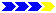Next page